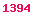The best books on Derivatives.
The best books on C++.
The best books on Java.

# Presentation: Proxy Simulation Schemes

Fries, Christian P.: Proxy Simulation Schemes for Generic Robust Monte Carlo Sensitivities and High-Accuracy Drift Approximation with Applications to the LIBOR Market Model. (PDF, 3 MB). (Version 1.0 - December 16th, 2005)

### Contents

• Monte Carlo Methods
• Temporal Discretisation Error
• Sensitivity Calculation
• Finite Differences
• Pathwise Differentiation
• Pathwise Differentiation (alternative view)
• Likelihood Ratio Method
• Malliavin Calculus
• Proxy Simulations Scheme Method
• Pricing & Sensitivity Calculation
• Densities & Weak Schemes
• Implementation
• Example: LIBOR Market Model
• Properties
• Degenerate Diffusion Matrix / Measure Equivalence
• Numerical Results
• Drift Approximations
• Sensitivity Calculations
• Appendix
• A Quadratic WKB Expansion for the LMM Transition Probability
• References

### Abstract

We consider a generic framework for generating likelihood ratio weighted Monte Carlo simulation paths, where we use one simulation scheme K° (proxy scheme) to generate realizations and then reinterpret them as realizations of another scheme K* (target scheme) by adjusting measure (via likelihood ratio) to match the distribution of K* such that

E( f(K*) | Ft ) = E( f(K°) • w | Ft ).

This is done numerically in every time step, on every path.

This makes the approach independent of the product (the function f) and even of the model, it only depends on the numerical scheme.

The approach is essentially a numerical version of the likelihood ratio method [Broadie & Glasserman, 1996] and Malliavin's Calculus [Fournie et al., 1999; Malliavin, 1997] reconsidered on the level of the discrete numerical simulation scheme.
Since the numerical scheme represents a time discrete stochastic process sampled on a discrete probability space the essence of the method may be motivated without a deeper mathematical understanding of the time continuous theory (e.g. Malliavin's Calculus).

The framework is completely generic and may be used for high accuracy drift approximations and the robust calculation of partial derivatives of expectations w.r.t. model parameters (i.e. sensitivities, aka. Greeks) by applying finite differences by reevaluating the expectation with a model with shifted parameters. We present numerical results using a Monte-Carlo simulation of the LIBOR Market Model for benchmarking.

Comments and suggestions welcomed at email@christian-fries.de.